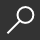## Basics of Modern Mathematical Statistics : Exercises and Solutions by Wolfgang Karl Härdle and Vladimir Spokoiny and Vladimir Panov

Overview -
The complexity of today's statistical data calls for modern mathematical tools. Many fields of science make use of mathematical statistics and require continuous updating on statistical technologies. Practice makes perfect, since mastering the tools makes them applicable. Our book of exercises and solutions offers a wide range of applications and numerical solutions based on R.
In modern mathematical statistics, the purpose is to provide statistics students with a number of basic exercises and also an understanding of how the theory can be applied to real-world problems.
The application aspect is also quite important, as most previous exercise books are mostly on theoretical derivations. Also we add some problems from topics often encountered in recent research papers.
The book was written for statistics students with one or two years of coursework in mathematical statistics and probability, professors who hold courses in mathematical statistics, and researchers in other fields who would like to do some exercises on math statistics.

local_shippingFor DeliveryIn Stock.
This item is Non-Returnable.
FREE Shipping for Club Members helpNew & Used Marketplace 3 copies from \$80.53

### Overview

The complexity of today's statistical data calls for modern mathematical tools. Many fields of science make use of mathematical statistics and require continuous updating on statistical technologies. Practice makes perfect, since mastering the tools makes them applicable. Our book of exercises and solutions offers a wide range of applications and numerical solutions based on R.
In modern mathematical statistics, the purpose is to provide statistics students with a number of basic exercises and also an understanding of how the theory can be applied to real-world problems.
The application aspect is also quite important, as most previous exercise books are mostly on theoretical derivations. Also we add some problems from topics often encountered in recent research papers.
The book was written for statistics students with one or two years of coursework in mathematical statistics and probability, professors who hold courses in mathematical statistics, and researchers in other fields who would like to do some exercises on math statistics.

This item is Non-Returnable.

### Details

• ISBN-13: 9783642368493
• ISBN-10: 3642368492
• Publisher: Springer
• Publish Date: November 2013
• Page Count: 185
• Dimensions: 9.21 x 6.14 x 0.5 inches
• Shipping Weight: 1.05 pounds

Series: Springer Texts in Statistics #122Related Categories